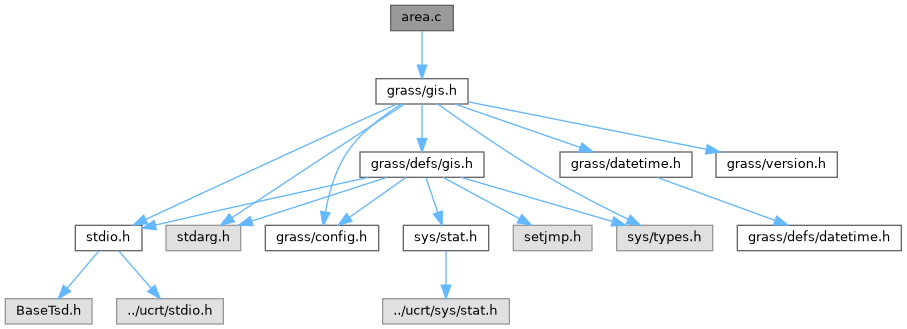GRASS GIS 8 Programmer's Manual  8.2.2dev(2023)-5c00b4e944
gis/area.c File Reference

GIS Library - Area calculation functions. More...

`#include <grass/gis.h>`
Include dependency graph for gis/area.c:Go to the source code of this file.

## Functions

int G_begin_cell_area_calculations (void)
Begin cell area calculations. More...

double G_area_of_cell_at_row (int row)
Cell area in specified row. More...

int G_begin_polygon_area_calculations (void)
Begin polygon area calculations. More...

double G_area_of_polygon (const double *x, const double *y, int n)
Area in square meters of polygon. More...

## Detailed Description

GIS Library - Area calculation functions.

(C) 2001-2009 by the GRASS Development Team

This program is free software under the GNU General Public License (>=v2). Read the file COPYING that comes with GRASS for details.

Definition in file gis/area.c.

## ◆ G_area_of_cell_at_row()

 double G_area_of_cell_at_row ( int row )

Cell area in specified row.

This routine returns the area in square meters of a cell in the specified row. This value is constant for planimetric grids and varies with the row if the projection is latitude-longitude.

Parameters
 row row number
Returns
cell area

Definition at line 87 of file gis/area.c.

References PROJECTION_LL, and st.

## ◆ G_area_of_polygon()

 double G_area_of_polygon ( const double * x, const double * y, int n )

Area in square meters of polygon.

Returns the area in square meters of the polygon described by the n pairs of x,y coordinate vertices. It is used both for planimetric and latitude-longitude projections.

You should call G_begin_polygon_area_calculations() function before calling this function.

Note: If the database is planimetric with the non-meter grid, this routine performs the required unit conversion to produce square meters.

Parameters
 x array of x coordinates y array of y coordinates n number of x,y coordinate pairs
Returns
area in square meters of the polygon

Definition at line 159 of file gis/area.c.

References G_ellipsoid_polygon_area(), G_planimetric_polygon_area(), PROJECTION_LL, st, and x.

## ◆ G_begin_cell_area_calculations()

 int G_begin_cell_area_calculations ( void )

Begin cell area calculations.

This routine must be called once before any call to G_area_of_cell_at_row(). It perform all inititalizations needed to do area calculations for grid cells, based on the current window "projection" field. It can be used in either planimetric projections or the latitude-longitude projection.

Returns
0 if the projection is not measurable (ie. imagery or xy)
1 if the projection is planimetric (ie. UTM or SP)
2 if the projection is non-planimetric (ie. latitude-longitude)

Definition at line 46 of file gis/area.c.

## ◆ G_begin_polygon_area_calculations()

 int G_begin_polygon_area_calculations ( void )

Begin polygon area calculations.

This initializes the polygon area calculation routines. It is used both for planimetric and latitude-longitude projections.

Returns
0 if the projection is not measurable (ie. imagery or xy)
1 if the projection is planimetric (ie. UTM or SP)
2 if the projection is non-planimetric (ie. latitude-longitude)

Definition at line 120 of file gis/area.c.